•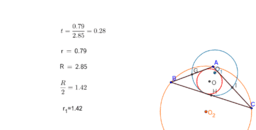### The ratio between R and r.

Activity

•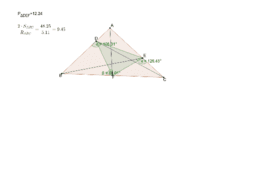### : Relationship among some elements of triangles

Activity

•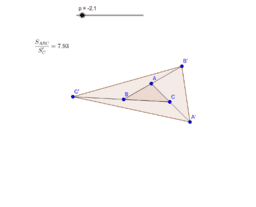### The ratio between the areas of two triangles

Activity

•### The ratio between R and r.

Activity

•### Relationship among some elements of triangles

Activity

•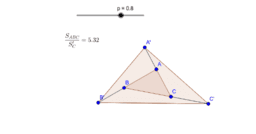### The ratio between the areas of two triangles

Activity

•### The ratio between R and r

Activity

•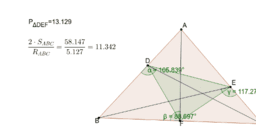### Relationship among some values sizes of triangles

Activity

•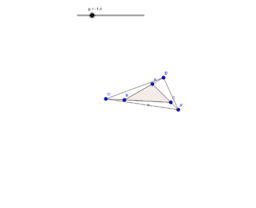### The ratio between the areas of two triangles

Activity

•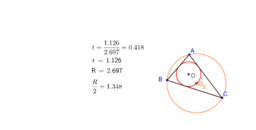### The ratio of the radii of the circles of a triangle

Activity

•### distance between two particular points in a triangle

Activity

•### The sum of the distances in a triangle

Activity

•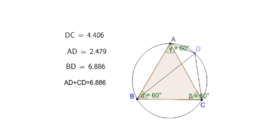### The sum of the lengths of the two chords

Activity

•### Angle bisection by means of a perpendicular to the side of a

Activity

•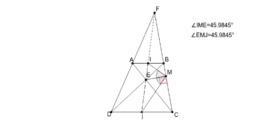### Angle bisection by means of a perpendicular to the side of a

Activity

•### The min value of the sum of the lengths of the hypotenuses

Activity

•### The max area of some triangle (stage b)

Activity

•### The max area of some triangle (stage a)

Activity

•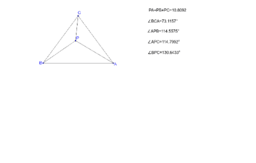### The sum of the distances is min (stage b)

Activity

•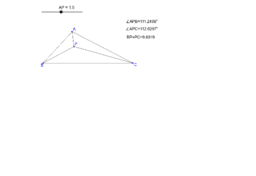### The sum of the distances is min (stage a)

Activity

•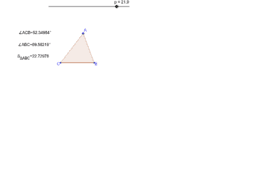### Triangle with a maximum area

Activity

•### sum of ligaments lengths of circuit in circuit

Activity

•### parallel line - cross-cutting of angles bisectors in triangl

Activity

•